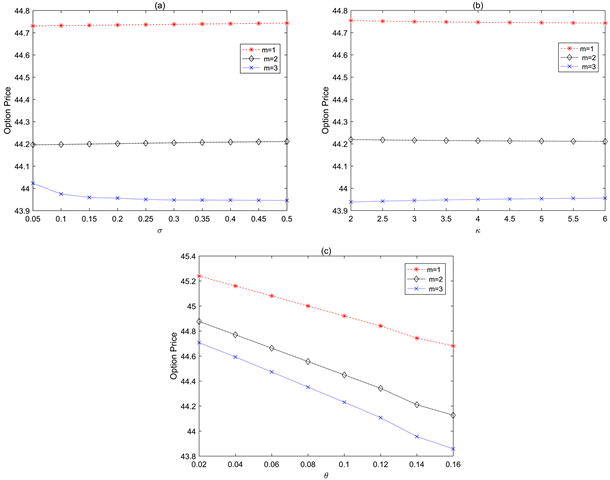# 随机波动率模型下离散几何平均亚式障碍期权定价Pricing of Discrete Geometric Average Asian Discrete Barrier Option under Stochastic Volatility Model

DOI: 10.12677/OJNS.2019.76054, PDF, HTML, XML, 下载: 435  浏览: 1,222

Abstract: In this paper, the pricing of Asian barrier options for discrete time scenarios based on the discrete geometric average of asset price is discussed under the model of Heston stochastic volatility which is discussed in the underlying asset price. Some stochastic analysis approaches such as the semi-martingale Itô formula, multidimensional federated characteristic functions, Girsanov theo-rem and Fourier inverse transform technique are to derive the pricing formula for the Asian dis-crete barrier call option. And finally, numerical examples are given by us, and the impacts of some parameters in stochastic volatility process on the values of the barrier option values are examined by this numerical example.

1. 引言

2. 市场模型与预备知识

$\left\{\begin{array}{l}\text{d}{S}_{t}={S}_{t}r\text{d}t+{S}_{t}\sqrt{{V}_{t}}\left(\rho \text{d}{W}_{t}+\sqrt{1-{\rho }^{2}}\text{d}{B}_{t}\right),\hfill \\ \text{d}{V}_{t}=\kappa \left(\theta -{V}_{t}\right)\text{d}t+\sigma \sqrt{{V}_{t}}\text{d}{W}_{t}.\hfill \end{array}$ (2.1)

${X}_{t}=\mathrm{ln}{S}_{t}$ ，由Itô公式，方程(2.1)变为

$\left\{\begin{array}{l}\text{d}{X}_{t}=\left(r-\frac{1}{2}{V}_{t}\right)\text{d}t+\sqrt{{V}_{t}}\left(\rho \text{d}{W}_{t}+\sqrt{1-{\rho }^{2}}\text{d}{B}_{t}\right),{X}_{0}={x}_{0},\hfill \\ \text{d}{V}_{t}=\kappa \left(\theta -{V}_{t}\right)\text{d}t+\sigma \sqrt{{V}_{t}}\text{d}{W}_{t},{V}_{0}={v}_{0}.\hfill \end{array}$ (2.2)

$\phi \left({u}_{1},{u}_{2},\cdots ,{u}_{m}\right)=\mathrm{exp}\left\{\underset{k=1}{\overset{m}{\sum }}i{u}_{k}h\left({t}_{k}\right)-\underset{k=1}{\overset{m}{\sum }}q\left({u}_{k}\right)j\left({t}_{k}\right)+\underset{k}{\overset{m}{\sum }}{B}_{k}+{A}_{m}{v}_{0}\right\},$ (2.3)

${A}_{k}=A\left({t}_{m-k+1}-{t}_{m-k},q\left({u}_{n-k+1}\right)+{A}_{k-1},p\left(\underset{j=m-k+1}{\overset{m}{\sum }}{u}_{j}\right)\right)$ ，且 ${A}_{0}=0$

$h\left(t\right)={x}_{0}+rt,j\left(t\right)={v}_{0}+\kappa \theta t$$p\left(u\right)=\left(\frac{1}{2}-\kappa \frac{\rho }{\sigma }-\frac{1}{2}iu\left(1-{\rho }^{2}\right)\right)iu$$q\left(u\right)=iu\frac{\rho }{\sigma }$

$A\left(\tau ,a,b\right)=\frac{da\left(1+{\text{e}}^{-d\tau }\right)-\left(1-{\text{e}}^{-d\tau }\right)\left(2b+\kappa a\right)}{d\left(1+{\text{e}}^{-d\tau }\right)-\left(1-{\text{e}}^{-d\tau }\right)\left(\kappa -{\sigma }^{2}a\right)},$ (2.4)

$B\left(\tau ,a,b\right)=\frac{\kappa \theta }{{\sigma }^{2}}\left(\left(\kappa -d\right)\tau +2\mathrm{ln}\frac{2d}{d\left(1+{\text{e}}^{-d\tau }\right)-\left(1-{\text{e}}^{-d\tau }\right)\left(\kappa -{\sigma }^{2}a\right)}\right),$ (2.5)

$\begin{array}{l}{C}_{e}\left(t,{x}_{0},{v}_{0},K,T\right)\\ ={S}_{0}\left\{\frac{1}{2}+\frac{1}{\pi }{\int }_{0}^{+\infty }\Re \left[\frac{{\text{e}}^{-iu\mathrm{ln}K}{\phi }_{1}\left(u\right)}{iu}\right]\text{d}u\right\}-K{\text{e}}^{-rT}\left\{\frac{1}{2}+\frac{1}{\pi }{\int }_{0}^{+\infty }\Re \left[\frac{{\text{e}}^{-iu\mathrm{ln}K}\phi \left(u\right)}{iu}\right]\text{d}u\right\},\end{array}$ (2.6)

3. 主要结果

${V}_{DOC}^{\left(m\right)}={E}^{P}\left[{\text{e}}^{-rT}{\left({S}_{T}-K\right)}^{+}{1}_{\left({S}_{{t}_{1}}>L,\cdots ,{S}_{T}>L\right)}\right],$ (3.1)

${V}_{DOC}^{\left(m\right)}={E}^{P}\left[{\text{e}}^{-rT}{\left({J}_{T}-K\right)}^{+}{1}_{\left({J}_{{t}_{1}}>L,\cdots ,{J}_{T}>L\right)}\right].$ (3.2)

$\begin{array}{c}{V}_{DOC}^{\left(m\right)}={S}_{0}\left\{\frac{1}{{2}^{m}}\left(1-m{t}^{{P}_{1}}\left(\mathrm{ln}L\right)+\frac{m\left(m-1\right)}{2}{t}^{{P}_{1}}\left(\mathrm{ln}L,\mathrm{ln}L\right)-{t}^{{P}_{1}}\left(\mathrm{ln}L,\mathrm{ln}L,\mathrm{ln}L\right)+\cdots \\ \begin{array}{c}\text{ }\\ \text{ }\end{array}+{\left(-1\right)}^{m}{t}^{{P}_{1}}\left(\mathrm{ln}L,\cdots ,\mathrm{ln}L\right)\right)\right\}-K{\text{e}}^{-rT}\left\{\frac{1}{{2}^{m}}\left(1-m{t}^{P}\left(\mathrm{ln}L\right)+\frac{m\left(m-1\right)}{2}{t}^{P}\left(\mathrm{ln}L,\mathrm{ln}L\right)\\ \begin{array}{c}\text{ }\\ \text{ }\end{array}-{t}^{P}\left(\mathrm{ln}L,\mathrm{ln}L,\mathrm{ln}L\right)+\cdots +{\left(-1\right)}^{m}{t}^{P}\left(\mathrm{ln}L,\cdots ,\mathrm{ln}L\right)\right)\right\},\end{array}$ (3.3)

${t}^{P}\left(\mathrm{ln}L,\cdots ,\mathrm{ln}L\right)=\frac{{\left(-2\right)}^{m}}{{\left(2\pi \right)}^{m}}\int \cdots {\int }_{{R}_{+}^{m}}{\Delta }_{{u}_{1}}\left[\cdots {\Delta }_{{u}_{m}}\left[\frac{{\phi }_{0}\left({u}_{1},\cdots ,{u}_{m}\right){\text{e}}^{-i\left({u}_{1}+\cdots +{u}_{m}\right)\mathrm{ln}L}}{\underset{k=1}{\overset{m}{\prod }}i{u}_{k}}\right]\right]\text{d}{u}_{1}\cdots \text{d}{u}_{m},$

${\Delta }_{{u}_{1}}\left[\cdots {\Delta }_{{u}_{m}}\left[\frac{\phi \left({u}_{1},\cdots ,{u}_{m}\right){\text{e}}^{-i\left({u}_{1}+\cdots +{u}_{m}\right)\mathrm{ln}L}}{\underset{k=1}{\overset{m}{\prod }}i{u}_{k}}\right]\right]=2{\Delta }_{{u}_{2}}\cdots {\Delta }_{{u}_{m}}\Re \left[\frac{\phi \left({u}_{1},\cdots ,{u}_{m}\right){\text{e}}^{-i\left({u}_{1}+\cdots +{u}_{m}\right)\mathrm{ln}L}}{\underset{k=1}{\overset{m}{\prod }}i{u}_{k}}\right],$

${\Delta }_{a}\left[f\left(a\right)\right]=f\left(a\right)+f\left(-a\right),$

${\phi }_{0}\left({u}_{1},\cdots ,{u}_{m}\right)={\text{e}}^{\left(\frac{i{u}_{1}}{2}+\frac{i{u}_{2}}{3}+\cdots +\frac{i{u}_{m}}{m+1}\right){x}_{0}}\phi \left(\frac{{u}_{1}}{2}\right)\phi \left(\frac{{u}_{2}}{3},\frac{{u}_{2}}{3}\right)\cdots \phi \left(\frac{{u}_{m}}{m+1},\cdots ,\frac{{u}_{m}}{m+1}\right),$

${\phi }_{1}\left({u}_{1},\cdots ,{u}_{m}\right)={\text{e}}^{-rT+\left(\frac{i{u}_{1}}{2}+\frac{i{u}_{2}}{3}+\cdots +\frac{i{u}_{m}-m}{m+1}\right){x}_{0}}\phi \left(\frac{{u}_{1}}{2}\right)\phi \left(\frac{{u}_{2}}{3},\frac{{u}_{2}}{3}\right)\cdots \phi \left(\frac{{u}_{m}-i}{m+1},\cdots ,\frac{{u}_{m}-i}{m+1}\right).$

$\begin{array}{c}{V}_{DOC}^{\left(1\right)}={E}^{P}\left\{{\text{e}}^{-rT}{\left({J}_{T}-K\right)}^{+}{1}_{\left({J}_{{t}_{1}}>L\right)}\right\}\\ ={E}^{P}\left\{{\text{e}}^{-rT}{J}_{T}{1}_{\left({J}_{{t}_{1}}>L\right)}\right\}-{E}^{P}\left\{{\text{e}}^{-rT}K{1}_{\left({J}_{{t}_{1}}>L\right)}\right\}\\ ={I}_{1}-{I}_{2},\end{array}$ (3.4)

${I}_{2}=K{\text{e}}^{-rT}P\left({J}_{{t}_{1}}>L\right)=K{\text{e}}^{-rT}P\left(\mathrm{ln}{J}_{{t}_{1}}>\mathrm{ln}L\right),$ (3.5)

$F\left(x\right)=P\left(X\ge x\right)=\frac{1}{2}+\frac{1}{\pi }{\int }_{0}^{+\infty }\Re \left[\frac{{\text{e}}^{-iux}f\left(u\right)}{iu}\right]\text{d}u,$ (3.6)

${I}_{2}=K{\text{e}}^{-rT}\left\{\frac{1}{2}+\frac{1}{\pi }{\int }_{0}^{+\infty }\Re \left[\frac{{\text{e}}^{-iu\mathrm{ln}L}{\phi }_{0}\left({u}_{1}\right)}{i{u}_{1}}\right]\text{d}{u}_{1}\right\},$ (3.7)

$\begin{array}{c}{\phi }_{0}\left({u}_{1}\right)={E}^{P}\left[\mathrm{exp}\left\{i{u}_{1}\mathrm{ln}{J}_{{t}_{1}}\right\}|{\mathcal{F}}_{t}\right]={E}^{P}\left[\mathrm{exp}\left\{\frac{i{u}_{1}}{2}\left(\mathrm{ln}{S}_{0}+\mathrm{ln}{S}_{{t}_{1}}\right)\right\}|{\mathcal{F}}_{t}\right]\\ ={\text{e}}^{\frac{i{u}_{1}}{2}{x}_{0}}{E}^{P}\left[\mathrm{exp}\left\{\frac{i{u}_{1}}{2}{X}_{{t}_{1}}\right\}|{\mathcal{F}}_{t}\right]={\text{e}}^{\frac{i{u}_{1}}{2}{x}_{0}}\phi \left(\frac{{u}_{1}}{2}\right).\end{array}$ (3.8)

$\frac{\text{d}{P}_{1}}{\text{d}P}\mid {\mathcal{F}}_{t}=\frac{{\text{e}}^{-rT}{J}_{T}}{{S}_{0}}.$

${I}_{1}={S}_{0}{P}_{1}\left({J}_{{t}_{1}}>L\right)={S}_{0}{P}_{1}\left(\mathrm{ln}{J}_{{t}_{1}}>\mathrm{ln}L\right)={S}_{0}\left\{\frac{1}{2}+\frac{1}{\pi }{\int }_{0}^{+\infty }\Re \left[\frac{{\text{e}}^{-iu\mathrm{ln}L}{\phi }_{1}\left({u}_{1}\right)}{i{u}_{1}}\right]\text{d}{u}_{1}\right\},$ (3.9)

$\begin{array}{c}{\phi }_{1}\left({u}_{1}\right)={E}^{{P}_{1}}\left[\mathrm{exp}\left\{i{u}_{1}\mathrm{ln}{J}_{{t}_{1}}\right\}|{\mathcal{F}}_{t}\right]={E}^{P}\left[\frac{{\text{e}}^{-rT}{J}_{T}}{{S}_{0}}{\text{e}}^{i{u}_{1}\mathrm{ln}{J}_{{t}_{1}}}|{\mathcal{F}}_{t}\right]\\ ={\text{e}}^{-rT-{x}_{0}}{E}^{P}\left[\mathrm{exp}\left\{\left(i{u}_{1}+1\right)\mathrm{ln}{J}_{{t}_{1}}\right\}|{\mathcal{F}}_{t}\right]={\text{e}}^{-rT+\frac{i{u}_{1}-1}{2}{x}_{0}}\phi \left(\frac{{u}_{1}-i}{2}\right).\end{array}$ (3.10)

$m=2$ 时，具有2个离散时间点 $0={t}_{0}<{t}_{1}<{t}_{2}=T$ ，根据风险中性定价原理得：

$\begin{array}{c}{V}_{DOC}^{\left(2\right)}={E}^{P}\left\{{\text{e}}^{-r\tau }{\left({J}_{T}-K\right)}^{+}{1}_{\left({J}_{{t}_{1}}>L,{J}_{T}>L\right)}\right\}\\ ={E}^{P}\left\{{\text{e}}^{-rT}{J}_{T}{1}_{\left({J}_{{t}_{1}}>L,{J}_{T}>L\right)}\right\}-{E}^{P}\left\{{\text{e}}^{-rT}K{1}_{\left({J}_{{t}_{1}}>L,{J}_{T}>L\right)}\right\}\\ ={I}_{1}-{I}_{2},\end{array}$ (3.11)

$\begin{array}{c}{I}_{2}=K{\text{e}}^{-rT}P\left(\mathrm{ln}{J}_{{t}_{1}}>\mathrm{ln}L,\mathrm{ln}{J}_{{t}_{2}}>\mathrm{ln}L\right)\\ =K{\text{e}}^{-rT}\left\{\frac{1}{4}+\frac{1}{2\pi }{\int }_{0}^{+\infty }\Re \left[\frac{{\text{e}}^{-i{u}_{1}\mathrm{ln}L}{\phi }_{0}\left({u}_{1},0\right)}{i{u}_{1}}\right]\text{d}{u}_{1}+\frac{1}{2\pi }{\int }_{0}^{+\infty }\Re \left[\frac{{\text{e}}^{-i{u}_{2}\mathrm{ln}L}{\phi }_{0}\left(0,{u}_{2}\right)}{i{u}_{2}}\right]\text{d}{u}_{2}\\ \text{\hspace{0.17em}}\text{\hspace{0.17em}}-\frac{1}{2{\pi }^{2}}\int {\int }_{{R}_{+}^{2}}\left\{\Re \left[\frac{{\text{e}}^{-i\left({u}_{1}+{u}_{2}\right)\mathrm{ln}L}{\phi }_{0}\left({u}_{1},{u}_{2}\right)}{{u}_{1}{u}_{2}}-\frac{{\text{e}}^{-i\left({u}_{1}-{u}_{2}\right)\mathrm{ln}L}{\phi }_{0}\left({u}_{1},-{u}_{2}\right)}{{u}_{1}{u}_{2}}\right]\right\}\text{d}{u}_{1}\text{d}{u}_{2}\right\},\end{array}$ (3.12)

$\begin{array}{c}{\phi }_{0}\left({u}_{1},{u}_{2}\right)={E}^{P}\left[\mathrm{exp}\left\{i{u}_{1}\mathrm{ln}{J}_{{t}_{1}}+i{u}_{2}\mathrm{ln}{J}_{T}\right\}|{\mathcal{F}}_{t}\right]\\ ={E}^{P}\left[\mathrm{exp}\left\{\frac{i{u}_{1}}{2}\left(\mathrm{ln}{S}_{0}+\mathrm{ln}{S}_{{t}_{1}}\right)+\frac{i{u}_{2}}{3}\left(\mathrm{ln}{S}_{0}+\mathrm{ln}{S}_{{t}_{1}}+\mathrm{ln}{S}_{{t}_{2}}\right)\right\}|{\mathcal{F}}_{t}\right]\\ ={\text{e}}^{\left(\frac{i{u}_{1}}{2}+\frac{i{u}_{2}}{3}\right){x}_{0}}{E}^{P}\left[\mathrm{exp}\left\{\frac{i{u}_{1}}{2}{X}_{{t}_{1}}+\frac{i{u}_{2}}{3}\left({X}_{{t}_{1}}+{X}_{{t}_{2}}\right)\right\}|{\mathcal{F}}_{t}\right]\\ ={\text{e}}^{\left(\frac{i{u}_{1}}{2}+\frac{i{u}_{2}}{3}\right){x}_{0}}\phi \left(\frac{{u}_{1}}{2}\right)\phi \left(\frac{{u}_{2}}{3},\frac{{u}_{2}}{3}\right),\end{array}$ (3.13)

$\begin{array}{c}{I}_{1}={S}_{0}{P}_{1}\left(\mathrm{ln}{J}_{{t}_{1}}>\mathrm{ln}L,\mathrm{ln}{J}_{T}>\mathrm{ln}L\right)\\ ={S}_{0}\left\{\frac{1}{4}+\frac{1}{2\pi }{\int }_{0}^{+\infty }\Re \left[\frac{{\text{e}}^{-i{u}_{1}\mathrm{ln}L}{\phi }_{1}\left({u}_{1},0\right)}{i{u}_{1}}\right]\text{d}{u}_{1}+\frac{1}{2\pi }{\int }_{0}^{+\infty }\Re \left[\frac{{\text{e}}^{-i{u}_{2}\mathrm{ln}L}{\phi }_{1}\left(0,{u}_{2}\right)}{i{u}_{2}}\right]\text{d}{u}_{2}\\ \text{\hspace{0.17em}}\text{\hspace{0.17em}}-\frac{1}{2{\pi }^{2}}\int {\int }_{{R}_{+}^{2}}\left\{\Re \left[\frac{{\text{e}}^{-i\left({u}_{1}+{u}_{2}\right)\mathrm{ln}L}{\phi }_{1}\left({u}_{1},{u}_{2}\right)}{{u}_{1}{u}_{2}}-\frac{{\text{e}}^{-i\left({u}_{1}-{u}_{2}\right)\mathrm{ln}L}{\phi }_{1}\left({u}_{1},-{u}_{2}\right)}{{u}_{1}{u}_{2}}\right]\right\}\text{d}{u}_{1}\text{d}{u}_{2}\right\},\end{array}$ (3.14)

$\begin{array}{c}{\phi }_{1}\left({u}_{1},{u}_{2}\right)={E}^{{P}_{1}}\left[\mathrm{exp}\left\{i{u}_{1}\mathrm{ln}{J}_{{t}_{1}}+i{u}_{2}\mathrm{ln}{J}_{T}\right\}|{\mathcal{F}}_{t}\right]\\ ={E}^{P}\left[\frac{{\text{e}}^{-rT}{J}_{T}}{{S}_{0}}\mathrm{exp}\left\{i{u}_{1}\mathrm{ln}{J}_{{t}_{1}}+i{u}_{2}\mathrm{ln}{J}_{T}\right\}|{\mathcal{F}}_{t}\right]\\ ={E}^{P}\left[\mathrm{exp}\left\{-rT+i{u}_{1}\mathrm{ln}{J}_{{t}_{1}}+\left(i{u}_{2}+1\right)\mathrm{ln}{J}_{T}\right\}|{\mathcal{F}}_{t}\right]/{S}_{0}\\ ={\text{e}}^{-rT+\left(\frac{i{u}_{1}}{2}+\frac{i{u}_{2}-2}{3}\right){x}_{0}}\phi \left(\frac{{u}_{1}}{2}\right)\phi \left(\frac{{u}_{2}-i}{3},\frac{{u}_{2}-i}{3}\right).\end{array}$ (3.15)

$\begin{array}{c}{V}_{DOC}^{\left(m\right)}={S}_{0}\left\{\frac{1}{{2}^{m}}\left(1-m{t}^{{P}_{1}}\left(\mathrm{ln}L\right)+\frac{m\left(m-1\right)}{2}{t}^{{P}_{1}}\left(\mathrm{ln}L,\mathrm{ln}L\right)-{t}^{{P}_{1}}\left(\mathrm{ln}L,\mathrm{ln}L,\mathrm{ln}L\right)+\cdots \\ \begin{array}{c}\text{ }\\ \text{ }\end{array}+{\left(-1\right)}^{m}{t}^{{P}_{1}}\left(\mathrm{ln}L,\cdots ,\mathrm{ln}L\right)\right)\right\}-K{e}^{-rT}\left\{\frac{1}{{2}^{m}}\left(1-m{t}^{P}\left(\mathrm{ln}L\right)\\ +\frac{m\left(m-1\right)}{2}{t}^{P}\left(\mathrm{ln}L,\mathrm{ln}L\right)-{t}^{P}\left(\mathrm{ln}L,\mathrm{ln}L,\mathrm{ln}L\right)+\cdots \\ +{\left(-1\right)}^{m}{t}^{P}\left(\mathrm{ln}L,\cdots ,\mathrm{ln}L\right)\right)\right\},\end{array}$ (3.16)

${t}^{{P}_{1}}\left(\mathrm{ln}L,\cdots ,\mathrm{ln}L\right)=\frac{{\left(-2\right)}^{m}}{{\left(2\pi \right)}^{m}}\int \cdots {\int }_{{R}_{+}^{m}}{\Delta }_{{u}_{1}}\left[\cdots {\Delta }_{{u}_{m}}\left[\frac{{\phi }_{1}\left({u}_{1},\cdots ,{u}_{m}\right){\text{e}}^{-i\left({u}_{1}+\cdots +{u}_{m}\right)\mathrm{ln}L}}{\underset{k=1}{\overset{m}{\prod }}i{u}_{k}}\right]\right]\text{d}{u}_{1}\cdots \text{d}{u}_{m},$

${t}^{P}\left(\mathrm{ln}L,\cdots ,\mathrm{ln}L\right)=\frac{{\left(-2\right)}^{m}}{{\left(2\pi \right)}^{m}}\int \cdots {\int }_{{R}_{+}^{m}}{\Delta }_{{u}_{1}}\left[\cdots {\Delta }_{{u}_{m}}\left[\frac{\phi \left({u}_{1},\cdots ,{u}_{m}\right){\text{e}}^{-i\left({u}_{1}+\cdots +{u}_{m}\right)\mathrm{ln}L}}{\underset{k=1}{\overset{m}{\prod }}i{u}_{k}}\right]\right]\text{d}{u}_{1}\cdots \text{d}{u}_{m},$

${\Delta }_{{u}_{1}}\left[\cdots {\Delta }_{{u}_{m}}\left[\frac{\phi \left({u}_{1},\cdots ,{u}_{m}\right){\text{e}}^{-i\left({u}_{1}+\cdots +{u}_{m}\right)\mathrm{ln}L}}{\underset{k=1}{\overset{m}{\prod }}i{u}_{k}}\right]\right]=2{\Delta }_{{u}_{2}}\cdots {\Delta }_{{u}_{m}}\Re \left[\frac{\phi \left({u}_{1},\cdots ,{u}_{m}\right){\text{e}}^{-i\left({u}_{1}+\cdots +{u}_{m}\right)\mathrm{ln}L}}{\underset{k=1}{\overset{m}{\prod }}i{u}_{k}}\right],$

${\Delta }_{a}\left[f\left(a\right)\right]=f\left(a\right)+f\left(-a\right),\text{\hspace{0.17em}}\text{\hspace{0.17em}}{\phi }_{1}\left({u}_{1},\cdots ,{u}_{m}\right)=\frac{{\text{e}}^{-rT}}{{S}_{0}}\phi \left({u}_{1},\cdots ,{u}_{m}-i\right).$

4. 数值结果与分析

$K=35,L=40,{t}_{0}=0,T=1,{t}_{i}=\left\{\frac{1}{m},\frac{2}{m},\cdots ,1\right\},\theta =0.1444,r=0.03,\kappa =6,{v}_{0}=0.15,\sigma =0.5,\rho =-0.7$Table 1. Comparison of the prices of Asian discrete obstacle options and European discrete obstacle options under the Heston modelFigure 1. Influence of parameters on option price

5. 结论

  Black, F. and Scholes, M. (1973) The Pricing of Options and Corporate Liabilities. Journal of Political Economy, 83, 637-654. https://doi.org/10.1086/260062  Merton, R.C. (1976) Option Pricing When Underlying Stock Re-turns Are Discontinuous. Journal of Financial Economics, 3, 125-144. https://doi.org/10.1016/0304-405X(76)90022-2  Kou, S. (2002) A Jump Diffusion Model for Option Pricing. Management Science, 48, 1086-1101. https://doi.org/10.1287/mnsc.48.8.1086.166  Heston, S.L. (1993) A Closed-Form Solution for Options with Stochastic Volatility with Applications to Bond and Currency Options. Review of Financial Studies, 6, 327-343. https://doi.org/10.1093/rfs/6.2.327  Hull, J.C. and White, A. (1987) The Pricing of Options on Assets with Stochastic Volatilities. Journal of Finance, 42, 281-300. https://doi.org/10.1111/j.1540-6261.1987.tb02568.x  Stein, E.M. and Stein, J.C. (1991) Stocks Price Distri-bution with Stochastic Volatility: An Analytic Application. Review of Financial Studies, 4, 281-300. https://doi.org/10.1093/rfs/4.4.727  Chen, R. and Scott, L.O. (1992) Pricing Interest Rate Options in a Two-Factor Cox-Ingersoll-Ross Model of the Term Structure. Review of Financial Studies, 5, 613-636. https://doi.org/10.1093/rfs/5.4.613  Bates, D.S. (1996) Jumps and Stochastic Volatility: Exchange Rate Pro-cesses Implicit in Deutsche Mark Options. Review of Financial Studies, 9, 69-107. https://doi.org/10.1093/rfs/9.1.69  邓国和, 杨向群. 随机波动率与双指数跳扩散组合模型的美式期权定价[J]. 应用数学学报, 2009, 32(2): 236-255.  Broadie, M., Glasserman, P. and Kou, S. (1997) A Continuity Cor-rection for Discrete Barrier Options. Mathematical Finance, 7, 325-349. https://doi.org/10.1111/1467-9965.00035  Fusai, G. and Recchioni, M.C. (2007) Analysis of Quadrature Methods for Pricing Discrete Barrier Options. Journal of Economic Dynamics and Control, 31, 826-860. https://doi.org/10.1016/j.jedc.2006.03.002  Griebsch, S. and Wystup, U. (2011) On the Valuation of Fader and Discrete Barrier Options in Heston’s Stochastic Volatility Model. Quantitative Finance, 11, 693-709. https://doi.org/10.1080/14697688.2010.503375  薛广明, 邓国和. 基于Bates模型的欧式离散障碍期权定价[J]. 华中师范大学学报: 自然科学版, 2018, 52(2): 164-171.  Duffie, D., Kan, R. and Singeton, K. (2000) Transform Analysis and Asset Pricing for Affine Jump-Diffusion. Econometrica, 68, 1343-1376. https://doi.org/10.1111/1468-0262.00164  Shephard, N.G. (1991) From Characteristic Function to Distribution Function: A Simple Framework for the Theory. Econometric Theory, 7, 519. https://doi.org/10.1017/S0266466600004746